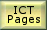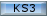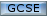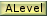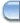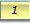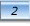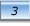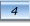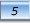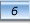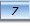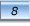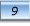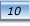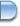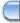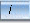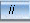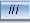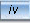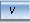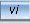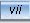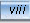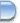There is quite a lot of work left to do on module 6.. for now here is

## An introduction to data flow modeling using SSADM notation.

1. Introduction to Data Flow Modelling
2. Components of a Data Flow Diagram, (SSADM notation)
3. Hints for Drawing Data Flow Diagrams
4. Electrical World - A Case Study in Analysis Techniques
5. Level 2 Data Flow Diagrams
6. WordWise Case Study
7. Decomposition to Level
8. Model Solution to Decomposition of WordWise

© LEV